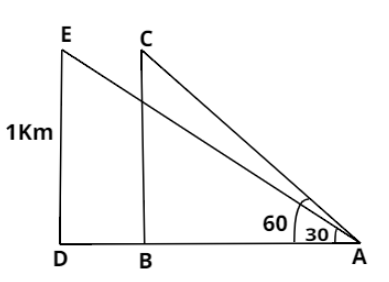Courses
Courses for Kids
Free study material
Offline Centres
MoreLast updated date: 04th Dec 2023
Total views: 381.6k
Views today: 4.81k

# An aeroplane flying horizontally 1 km above the ground is observed at an angle of ${60^ \circ }$. If after 10 seconds the elevation is observed to be ${30^ \circ }$, find the uniform speed of the aeroplane.Verified
381.6k+ views
Hint: Consider different triangles of different positions of the aeroplane. The distance travelled by the aeroplane in 10 seconds will be the difference between the different positions.In the above figure, C is the initial position of the aeroplane. Its height above the ground is 1 km. Initial angle of elevation is ${60^ \circ }$. Then in triangle ABC, we have:
$\Rightarrow \tan {60^ \circ } = \dfrac{{BC}}{{AB}}, \\ \Rightarrow \sqrt 3 = \dfrac{1}{{AB}}, \\ \Rightarrow AB = \dfrac{1}{{\sqrt 3 }} .....(i) \\$
From the figure, $CE = BD$
Let the uniform speed of the aeroplane is $v{\text{ km/hr}}$. It moves horizontally for 10 seconds and reaches point E. We know, Distance $=$Speed $\times$time. Applying this, we’ll get:
$\Rightarrow CE = v \times \dfrac{{10}}{{60 \times 60}}$
From the figure, $CE = BD$. So, we have:
$\Rightarrow BD = v \times \dfrac{{10}}{{60 \times 60}} .....(ii)$
From the figure, we have:
$\Rightarrow AD = AB + BD$
Putting the values of AB and BD from equations $(i)$ and $(ii)$ respectively, we’ll get:
$\Rightarrow AD = \dfrac{1}{{\sqrt 3 }} + v \times \dfrac{{10}}{{60 \times 60}} .....(iii)$
The angle of elevation of the aeroplane now is ${30^ \circ }$. Thus, in triangle ADE, we have:
$\Rightarrow \tan {30^ \circ } = \dfrac{{DE}}{{AD}}, \\ \Rightarrow \dfrac{1}{{\sqrt 3 }} = \dfrac{1}{{AD}}, \\ \Rightarrow AD = \sqrt 3 \\$
Now putting the value of AD from equation $(iii)$, we’ll get:
$\Rightarrow \dfrac{1}{{\sqrt 3 }} + v \times \dfrac{{10}}{{60 \times 60}} = \sqrt 3 , \\ \Rightarrow \dfrac{v}{{6 \times 60}} = \sqrt 3 - \dfrac{1}{{\sqrt 3 }}, \\ \Rightarrow \dfrac{v}{{60 \times 2 \times 3}} = \dfrac{2}{{\sqrt 3 }}, \\ \Rightarrow v = 60 \times 2 \times \sqrt 3 \times 2, \\ \Rightarrow v = 240\sqrt 3 \\$
Thus the speed of the aeroplane is $240\sqrt 3$ km/hr.

Note: The question can be solved in this way only if the aeroplane is moving horizontally. If it is moving at any other angle, the triangles formed will be different than this.